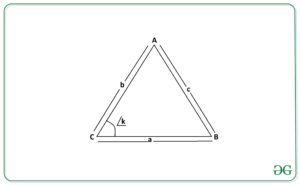# Area of Triangle using Side-Angle-Side (length of two sides and the included angle)

Given two integers A, B representing the length of two sides of a triangle and an integer K representing the angle between them in radian, the task is to calculate the area of the triangle from the given information.

Examples:

Input: a = 9, b = 12, K = 2
Output: 49.1
Explanation:
Area of triangle = 1 / 2 * (9 * 12 * Sin 2) = 35.12

Input: A = 2, B = 4, K = 1
Output: 3.37

## Recommended: Please try your approach on {IDE} first, before moving on to the solution.

Approach:
Consider the following triangle ABC with sides A, B, C, and an angle K between sides A and B.Then, the area of the triangle can be calculated using the Side-Angle-Side formula:Below is the implementation of the above approach:

## C++

 // C++ program to calculate   // the area of a triangle when   // the length of two adjacent   // sides and the angle between   // them is provided   #include  using namespace std;     float Area_of_Triangle(int a, int b, int k)   {       float area = (float)((1 / 2.0) *                             a * b * (sin(k)));          return area;   }      // Driver Code   int main()  {       int a = 9;       int b = 12;       int k = 2;          // Function Call       float ans = Area_of_Triangle(a, b, k);          // Print the final answer       cout << ans << endl;  }      // This code is contributed by Ritik Bansal

## Java

 // Java program to calculate  // the area of a triangle when   // the length of two adjacent   // sides and the angle between  // them is provided  class GFG{     // Function to return the area of   // triangle using Side-Angle-Side  // formula   static float Area_of_Triangle(int a, int b,                                       int k)  {      float area = (float)((1 / 2.0) *                         a * b * Math.sin(k));         return area;  }     // Driver Code   public static void main(String[] args)  {      int a = 9;      int b = 12;      int k = 2;         // Function Call      float ans = Area_of_Triangle(a, b, k);         // Print the final answer      System.out.printf("%.1f",ans);  }  }     // This code is contributed by sapnasingh4991

## Python3

 # Python3 program to calculate  # the area of a triangle when   # the length of two adjacent   # sides and the angle between  # them is provided     import math      # Function to return the area of   # triangle using Side-Angle-Side  # formula   def Area_of_Triangle(a, b, k):          area =(1 / 2) * a * b * math.sin(k)         return area      # Driver Code   a = 9 b = 12 k = 2    # Function Call   ans = Area_of_Triangle(a, b, k)      # Print the final answer   print(round(ans, 2))   

## C#

 // C# program to calculate  // the area of a triangle when   // the length of two adjacent   // sides and the angle between  // them is provided  using System;  class GFG{     // Function to return the area of   // triangle using Side-Angle-Side  // formula   static float Area_of_Triangle(int a, int b,                                       int k)  {      float area = (float)((1 / 2.0) *                     a * b * Math.Sin(k));         return area;  }     // Driver Code   public static void Main(String[] args)  {      int a = 9;      int b = 12;      int k = 2;         // Function Call      float ans = Area_of_Triangle(a, b, k);         // Print the readonly answer      Console.Write("{0:F1}", ans);  }  }     // This code is contributed by sapnasingh4991

Output:

49.1


Time Complexity: O(1)
Auxiliary Space: O(1)

Attention reader! Don’t stop learning now. Get hold of all the important DSA concepts with the DSA Self Paced Course at a student-friendly price and become industry ready.

My Personal Notes arrow_drop_upCheck out this Author's contributed articles.

If you like GeeksforGeeks and would like to contribute, you can also write an article using contribute.geeksforgeeks.org or mail your article to contribute@geeksforgeeks.org. See your article appearing on the GeeksforGeeks main page and help other Geeks.

Please Improve this article if you find anything incorrect by clicking on the "Improve Article" button below.

Article Tags :
Practice Tags :

Be the First to upvote.

Please write to us at contribute@geeksforgeeks.org to report any issue with the above content.